# DAV Class 4 Maths Chapter 4 Worksheet 1 Solutions

The DAV Class 4 Maths Book Solutions and DAV Class 4 Maths Chapter 4 Worksheet 1 Solutions of Division offer comprehensive answers to textbook questions.

## DAV Class 4 Maths Ch 4 WS 1 Solutions

Question 1.
(a) 98 by 8Quotient = 12
Remainder = 2
Divisor = 8
Dividend = 98

check:
Divisor × Quotient + Remainder = Dividend
L.H.S. = 8 × 12 + 2
= 96 + 2
= 98
R.H.S. (Dividend) = 98
∴ L.H.S. = R.H.S.

(b) 87 by 9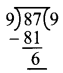Quotient = 9
Remainder = 6
Divisor = 9
Dividend = 87

To check:
Divisor × Quotient + Remainder = Dividend
L.H.S. = 9 × 9 + 6
= 81 + 6
= 87
R.H.S. = 87
∴ L.H.S. = R.H.S.

(c) 725 by 10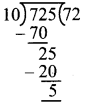Quotient = 72
Remainder = 10
Divisor = 5
Dividend = 725
To check:
Divisor × Quotient + Remainder = Dividend
L.H.S. = 10 × 72 + 5
= 720 + 5
= 725
R.H.S. = 725
∴ L.H.S. = R.H.S.

(d) 547 by 7To check:
Divisor × Quotient + Remainder = Dividend
L.H.S. = 7 × 78 + 1
= 546 + 1
= 547
R.H.S. = 547
∴ L.H.S. = R.H.S.

(e) 918 by 10Quotient = 91
Remainder = 8
Divisor =10
Dividend = 918

To check:
Divisor × Quotient + Remainder = Dividend
L.H.S. = 10 × 91 + 8
= 910 + 8
= 918
R.H.S. = 918
∴ L.H.S. = R.H.S.

(f) 132 by 6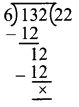Quotient = 22
Remainder = 0
Divisor = 6
Dividend = 132

To check:
Divisor × Quotient + Remainder = Dividend
L.H.S. = 6 × 22 + 0
= 132
R.H.S. = 132
∴ L.H.S. = R.H.S.Question 2.
Keeping the relation between divisor, quotient remainder and dividend in mind, find the missing numbers.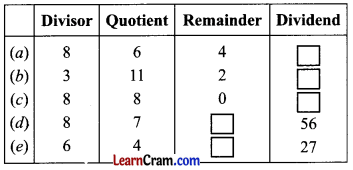(a) Dividend = Divisor × Q + R
= 8 × 6 + 4
= 48 + 4
= 52

(b) Dividend = 3 × 11 + 2
= 33 + 2
= 35

(c) Dividend = 8 × 8 + 0
= 64

(d) Dividend = Divisor × Q + R
56 = 8 × 7 + R
56 = 56 + R
0 = R

(e) Dividend = Divisor × Q + R
27 = 6 × 4 + R
27 – 24 = R
R = 3

### DAV Class 4 Maths Chapter 4 Worksheet 1 Notes

• For doing division one must be well versed with the multiplication tables.
• Dividend = Divisor x Quotient + Remainder.
• We always start division from the extreme left of the dividend.
• When a number is divided by 10, 100 or 1000, the quotient is obtained by removing the number of digit present at right as that of the zeroes i.e. 1, 2 or 3 digits respectively. The digit or digits removed is the remainder.Review Exercise

Question 1.
Divide and find the quotient and remainder.
(a) 84 ÷ 4Quotient = 21
Remainder = 0

(b) 984 ÷ 9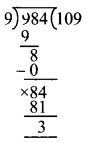Quotient = 109
Remainder = 3

(c) 786 ÷ 10Quotient = 78
Remainder = 6

(d) 465 ÷ 8Quotient = 58
Remainder = 1

(e) 720 ÷ 10Quotient = 72
Remainder = 0

(f) 864 ÷ 8Quotient = 108
Remainder = 0

(g) 118 ÷ 6Quotient = 19
Remainder = 4

(h) 226 ÷ 4Quotient = 56
Remainder = 2

(i) 643 ÷ 7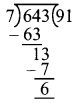Quotient = 91
Remainder = 6Question 2.
Divide orally using multiplication tables.
(a) 15 ÷ 55 × 1 = 5
5 × 2 = 10
5 × 3 = 15

(b) 56 ÷ 8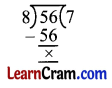8 × 1 = 8
8 × 2 = 16
8 × 3 = 24
8 × 4 = 32
8 × 5 = 40
8 × 6 = 48
8 × 7 = 56

(c) 70 ÷ 10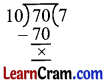10 × 1 = 10
10 × 2 = 20
10 × 3 = 30
10 × 4 = 40
10 × 5 = 50
10 × 6 = 60
10 × 7 = 70

(d) 63 ÷ 99 × 1 = 9
9 × 2 = 18
9 × 3 = 24
9 × 4 = 36
9 × 5 = 45
9 × 6 = 54
9 × 7 = 63

(e) 28 ÷ 77 × 1 = 7
7 × 2 = 14
7 × 3 = 21
7 × 4 = 28

(f) 36 ÷ 66 × 1 = 6
6 × 2 = 12
6 × 3 = 18

Question 3.
Fill in the blanks.
(a) 17 ÷ 1 = ____
(b) 0 ÷ 8 = ____
(c) 32 ÷ 32 = ____
(d) 9 ÷ ____ = 9
(e) ____ ÷ 6 = 0
(f) ____ ÷ 1 = 18